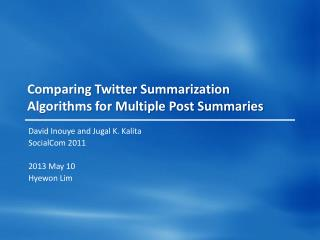# Comparing Twitter Summarization Algorithms for Multiple Post Summaries - PowerPoint PPT PresentationDownload PresentationComparing Twitter Summarization Algorithms for Multiple Post Summaries

Comparing Twitter Summarization Algorithms for Multiple Post Summaries
Download Presentation## Comparing Twitter Summarization Algorithms for Multiple Post Summaries

- - - - - - - - - - - - - - - - - - - - - - - - - - - E N D - - - - - - - - - - - - - - - - - - - - - - - - - - -
##### Presentation Transcript

1. Comparing Twitter Summarization Algorithms for Multiple Post Summaries David Inouye and Jugal K. Kalita SocialCom 2011 2013 May 10 Hyewon Lim

2. Outline • Introduction • Related Work • Problem Definition • Selected Approaches for Twitter Summaries • Experimental Setup • Results and Analysis • Conclusion

3. Introduction • Motivation of the summarizer

4. Introduction • Prior work • “A torch extinguished: Ted Kennedy dead at 77.”“A legend gone: Ted Kennedy died of brain cancer.”“Ted Kennedy was a leader.”“Ted Kennedy died today.” B. Sharifi et al., “Automatic Summarization of Twitter Topics”

5. Introduction • Prior work (cont.) • “A torch extinguished: Ted Kennedy dead at 77.”“A legend gone: Ted Kennedy died of brain cancer.”“Ted Kennedy was a leader.”“Ted Kennedy died today.” Best final summary: Ted Kennedy died B. Sharifi et al., “Automatic Summarization of Twitter Topics”

6. Introduction • We create summaries that contain multiple posts • Several sub-topics or themes in a specified topic

7. Outline • Introduction • Related Work • Problem Definition • Selected Approaches for Twitter Summaries • Experimental Setup • Results and Analysis • Conclusion

8. Related Work • Text summarization • Reduce the amount of content to read • Reduce the number of features required for classifying or clustering • Multi-document summarization • Potential redundancy • Algorithms • SumBasic, Centroid, LexRank, TextRank, MEAD, …

9. Related Work • SumBasic • Centroid “A torch extinguished: Ted Kennedy dead at 77.”“A legend gone: Ted Kennedy died of brain cancer.”“Ted Kennedy was a leader.”“Ted Kennedy died today.” Ted Kennedy died (D. R. Radev et al., “Centroid-based summarization of multiple documents”)

10. Related Work • LexRank • Adjacencymatrix for computing the relative importance of sentences • TextRank • Find the most highly ranked sentences using the PageRank Compatibility of systems of linear constraints over the set of natural numbers. Criteria of compatibility of a system of linear Diophantine equations, strict inequations, and nonstrictinequations are considered. Upper bounds for components of a minimal set of solutions and algorithms of construction of minimal generating sets of solutions for all types of systems are given. These criteria and the corresponding algorithms for constructing a minimal supporting set of solutions can be used in solving all the considered types systems and systems of mixed types.

11. Outline • Introduction • Related Work • Problem Definition • Selected Approaches for Twitter Summaries • Experimental Setup • Results and Analysis • Conclusion

12. Problem Definition • Given • A topic keyword or phrase T • Length k for the summary • Output • A set of representative posts S with a cardinality of ksuch that1) ∀s ∈ S, T is in the text of s2) ∀si, ∀sj∈ S, si≁ sj

13. Selected Approaches for Twitter Summaries • TF-IDF (Term frequency) * (Inverse document frequency) • A microblog post is not a traditional document • Define a single document that encompass all the posts => IDF↓ • Define each post as a document => TF↓ A A A…….A……… ……………A… …...................... ………………… …….A………… ………………… A

14. Selected Approaches for Twitter Summaries • Hybrid TF-IDF • Define a document as a single post • Computing the term frequencies • Assume the document is the entire collection of posts • Select the top k most weighted posts • Cosine similarity for avoiding redundancy

15. Selected Approaches for Twitter Summaries • Cluster summarizer • Cluster the tweets into k clusters based on a similarity measure • Summarize each cluster by picking the most weighted post • Bisecting k-means++ algorithm • Bisecting k-means • k-means++ • Chooses the next centroidci, selecting ci = v’ ∈ V with probability

16. Selected Approaches for Twitter Summaries • k-means++ Outlier problem k-means k-means++ http://blog.sragent.pe.kr/

17. Selected Approaches for Twitter Summaries • Algorithms to compare results • Baseline • Random summarizer • Most recent summarizer • SumBasic • Depends only on the frequency of words • MEAD • Comparison between the more structured document domain and Twitter • Graph-based method • LexRank • TextRank

18. Outline • Introduction • Related Work • Problem Definition • Selected Approaches for Twitter Summaries • Experimental Setup • Results and Analysis • Conclusion

19. Experimental Setup • Data collection • 5 consecutive days • Top ten currently trending topics every day • Approximately 1500 tweets for each topic • ROUGE • Automated summary vs. manual summaries • Choice of k

20. Results and Analysis • Average F-measure, precision and recall

21. Results and Analysis • Average score for human evaluation

22. Results and Analysis • Paired two-sided T-test

23. Outline • Introduction • Related Work • Problem Definition • Selected Approaches for Twitter Summaries • Experimental Setup • Results and Analysis • Conclusion

24. Conclusion • The best techniques for summarizing Twitter topics • Simple word frequency • Redundancy reduction • Simple algorithms seem to perform well • Not clear that added complexity will improve the quality of the summaries • Extension • Extrinsic evaluations (e.g., user survey) • Dynamically discovering a good value for k for k-means • Detect named entities and events in the documents